# CREST Reasoning Olympiad Class 1 Sample Papers

## Syllabus:

Section 1: Analogy, Geometrical Shapes, Grouping of Figures, Embedded Figures, Odd One Out, Pattern, Measuring Units, Ranking Test, Spatial Understanding.

Achievers Section: Higher Order Thinking Questions - Syllabus as per Section 1

 Q.1 Select one among the following that will complete the given sequence: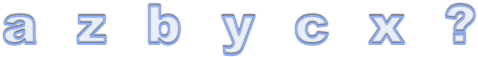Q.2 Which among the following will replace the question mark?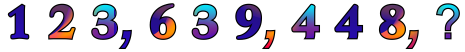Q.3 What is the position of the pumpkin from the right?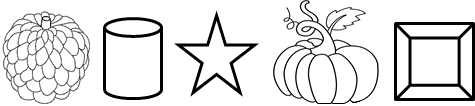Q.4 Neha has 10 chocolates. She gives 2 chocolates to Pooja and 2 to Sohan. Roopali gives Neha 5 chocolates. How many chocolates does Neha have?
 Q.5 Which figure/pattern/shape comes next?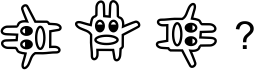Q.6 There is a certain relationship between the pair of figures given on either side of (::) identify the relationship of given pair and find the matching term.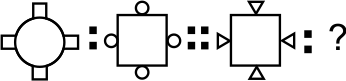Q.7 Which object is same as the given figure?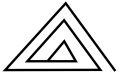Q.8 Given below is an image. Which among the following figure is related to the given image?Q.9 If there are 11 students in a group project and your position is 6th from the start, then what is your position from the last?
 Q.10 There is a certain relationship between the pair of figures given on either side of (::) identify the relationship of given pair and find the matching term.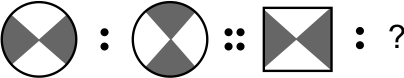Sample PDF of CREST Reasoning Olympiad for Class 1: